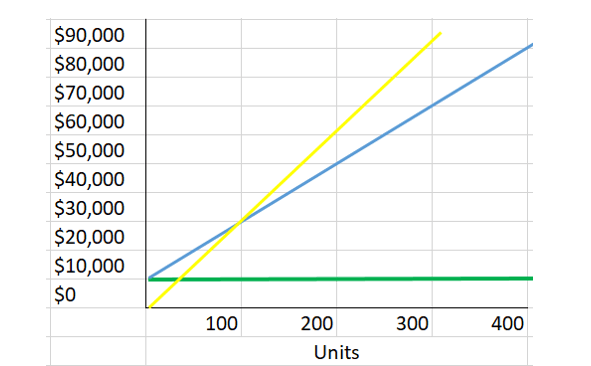CVP Graph

What is a CVP Graph?

Definition: The cost volume profit graph, which is abbreviated as the CVP graph, illustrates the relationship between the costs of production and sales.

What does CVP Graph mean?

The purpose of making a CVP graph is to help a company determine the impact that a change in sales volumes has on cost and profits.

It is necessary to make certain assumptions when preparing a CVP graph:

• Fixed costs remain constant regardless of the level of production.
• The company is able to sell each unit that it produces.
• The selling price of each unit is the same.
• Each additional unit of production results in the same increase in variable costs.

One of the key benefits that a CVP graph provides is that it tells a company about its breakeven point. This allows a firm to plan its production and sales activities.

The CVP graph also puts costs into focus. It highlights fixed costs as well as variable costs.

Example of CVP Graph

The following CVP graph represents Zephyr Corporation’s costs and production levels:The vertical axis denotes dollar amounts and the horizontal axis represents units of production.

The thick green line at the \$10,000 level represents fixed costs. This does not change with the level of production. It remains constant at \$10,000 even if there is no production.

The blue line is the total cost line. When there is zero production, total costs are at \$10,000. The total costs gradually rise with the increase in production.

The yellow line represents sales. At a 100 units of production, when total costs are at \$30,000, breakeven is achieved. After this point, Zephyr starts making profits.

Summary

This simple graphical representation that presents data about fixed costs, variable costs, and sales can be a useful tool for determining the company’s future profitability.

=